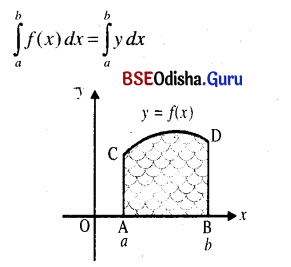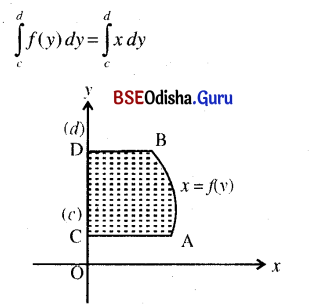# CHSE Odisha Class 12 Math Notes Chapter 10 Area Under Plane Curves

Odisha State Board CHSE Odisha Class 12 Math Notes Chapter 10 Area Under Plane Curves will enable students to study smartly.

## CHSE Odisha 12th Class Math Notes Chapter 10 Area Under Plane CurvesArea of the portion bounded by x-axis the curve
y = f(x) and two ordinates at x = a and x = b.= Area of the portion bounded by y-axis,
the curve x = f(y) and two abscissa at y = c and y = d.

Area between two curves y = f(x), y g(x) with g(x) < f(x) in [a, b] and between two ordinates
x = a and x = b is given by $$\int_a^b$${f(x) – g(x)}dx